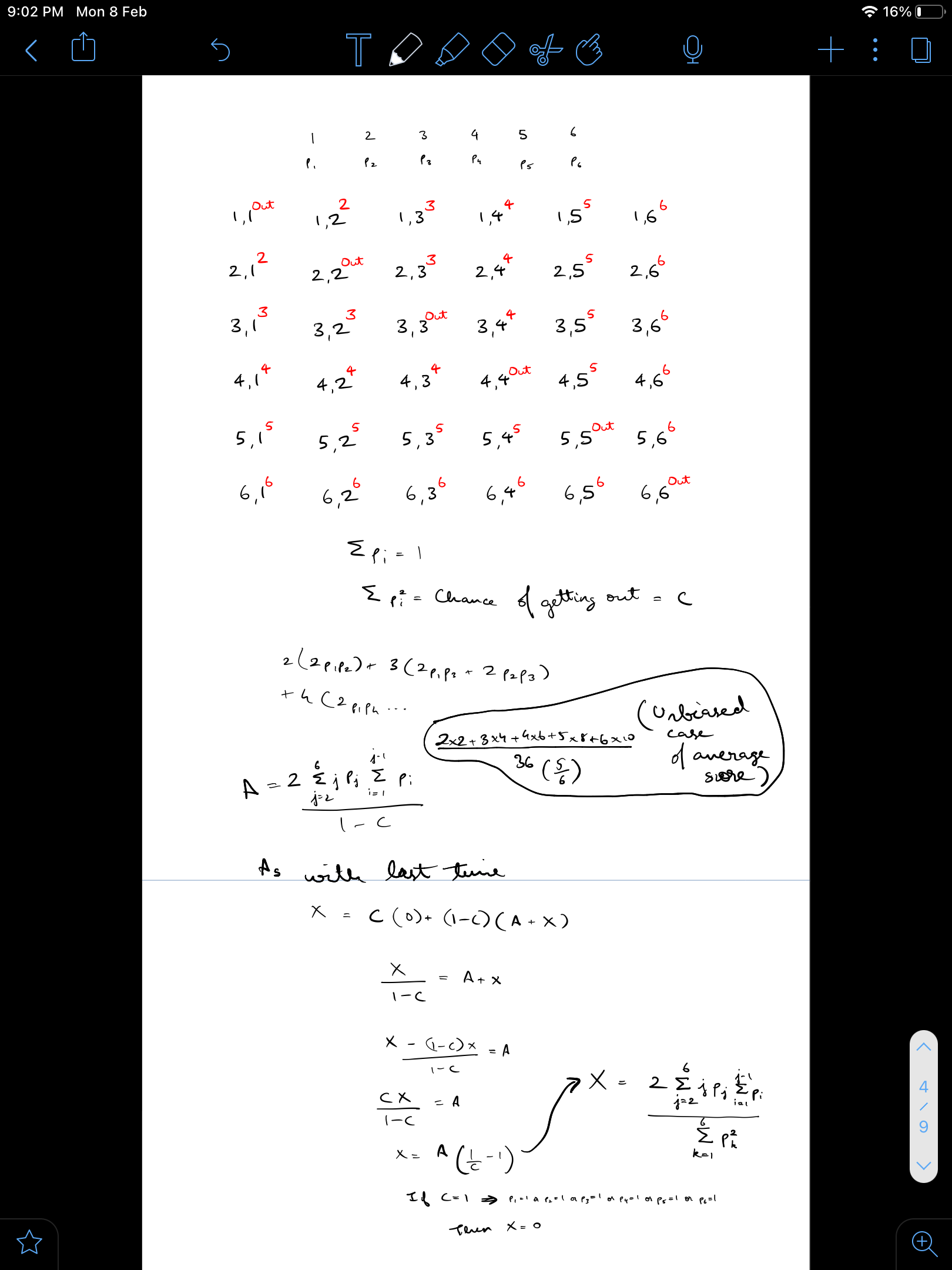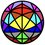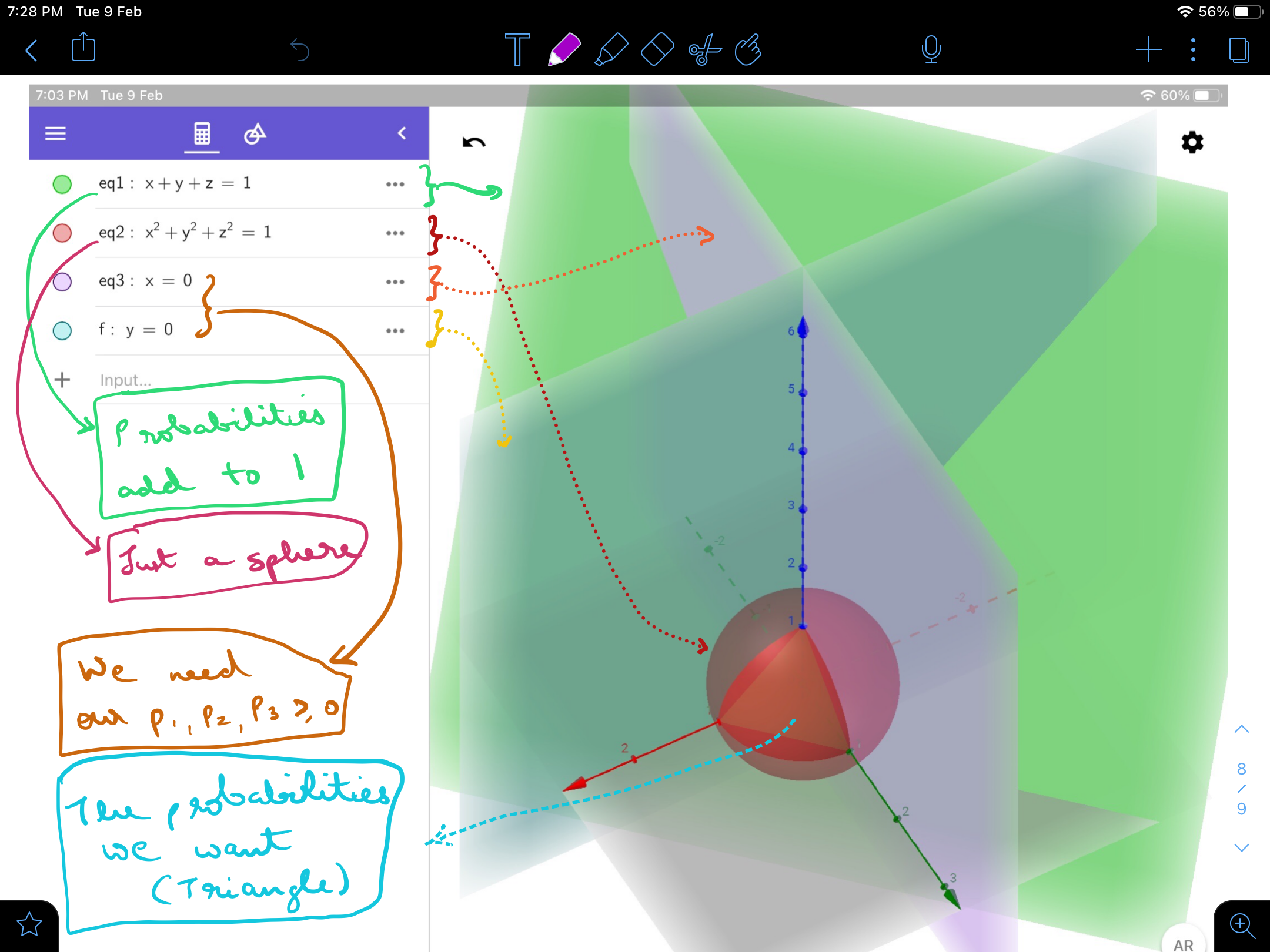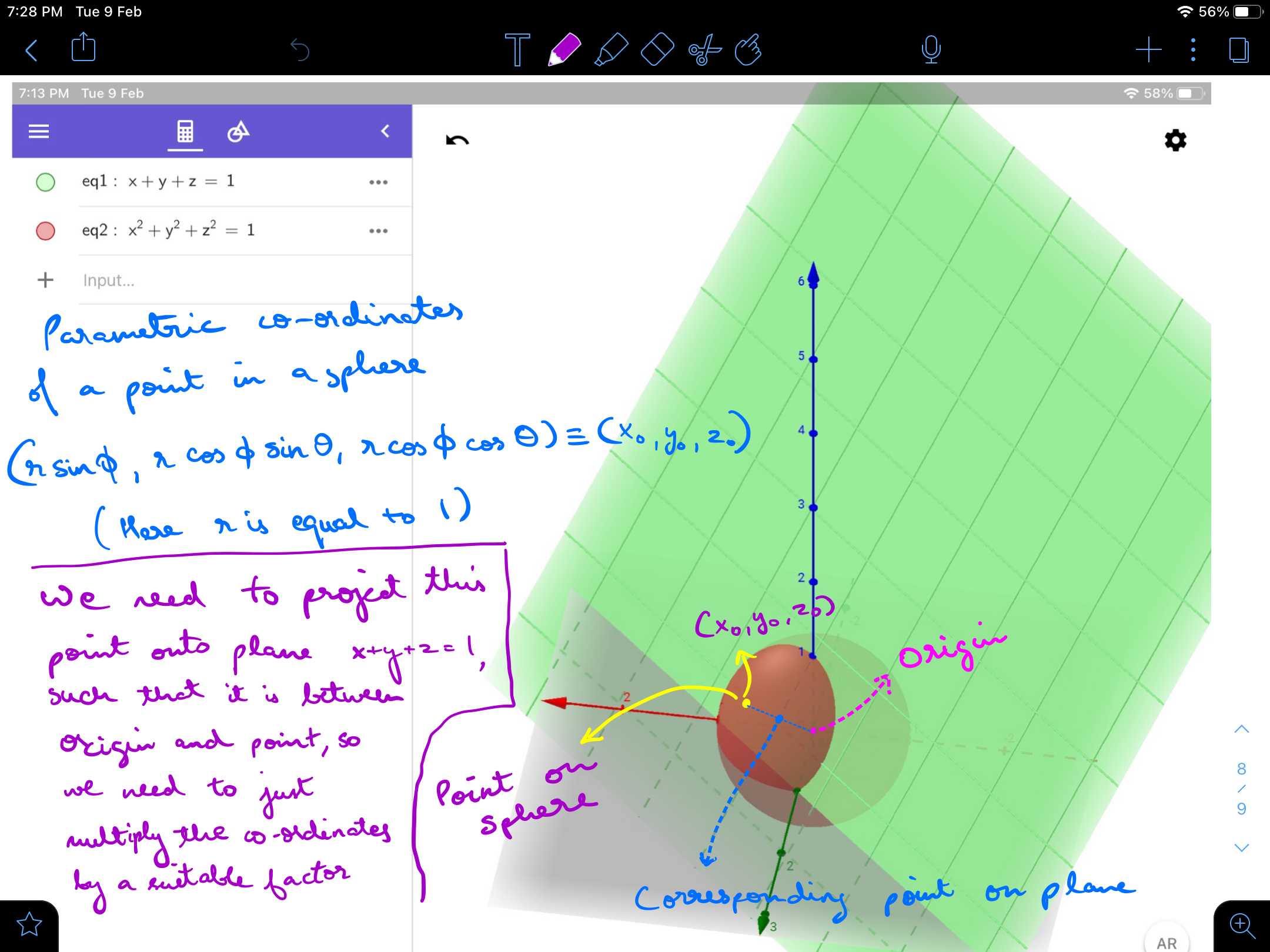# Rollin a biased die

In my most recent, non-reported, second problem (the first one was heavily reported two-four years ago which discouraged me from making problems), there was a change made to it by the staff which got me thinking (they added that the die were unbiased), what if the die were biased but equally, can Alex get a higher expected score (Xela uses a copy of the die Alex uses so the probabilities of both die are equal), turns out it’s true, he can get a higher expected score but since I do not know how to do multi-variable calculus, I couldn’t find the upper bound

Defining some terminology: $p_i$ = Probability of i appearing on the dice, $i ∈ {1,2,3,4,5,6}$

Chance of getting out $= C = \displaystyle \sum_{i=1}^6 p_i^2$

Average score per round $= A = \frac2 × \sum_{j=2}^6 j× p_j \sum_{i=1}^{j-1} p_i}1-C}$

Expected score $= X = A × (\frac{1}{C} -1) = \frac2 × \sum_{j=2}^6 j× p_j \sum_{i=1}^{j-1} p_i}C}$

Here’s a picture to help you see how I got these formulaeUsing this program I was able to find somewhat where the answer should lie

  1 2 3 4 5 6 7 8 9 10 11 12 13 14 15 16 17 18 19 20 21 22 23 24 from random import random from math import sin,cos,pi from itertools import permutations Xmax=0 no=0 while True: no+=1 a=[pi/2*random() for i in range(5)]#contains angles of the six dimensional sphere h=[sin(a),cos(a)*sin(a),cos(a)*cos(a)*sin(a),cos(a)*cos(a)*cos(a)*sin(a),cos(a)*cos(a)*cos(a)*cos(a)*sin(a),cos(a)*cos(a)*cos(a)*cos(a)*cos(a)]#contains the six co-ordinates of the sphere tot=h+h+h+h+h+h#reducing factor to get the points on the sphere to land on the cube h.sort() p=[h[i]/tot for i in range(6)]#points on the six dimensional cube, the required probabilities C=p**2+p**2+p**2+p**2+p**2+p**2 sum2=0 for j in range(1,6): sum1=0 for i in range(j): sum1+=p[i] sum2+=(j+1)*p[j]*sum1 A=sum2*2/(1-C) X=sum2*2/(C) if X>Xmax: Xmax=X print(X,p) 

After more than 1 billion random checks, the highest expected score I reached was 23.752142249471124 with $p_1=$ 0.1487492948991272, $p_2=$ 0.14899405525187628, $p_3=$ 0.154656034331149, $p_4=$ 0.16515216949050074, $p_5=$ 0.1808399982323611, $p_6=$ 0.20160844779498574, which is not very far from the 23.33… obtainable from an unbiased die

If there are any mistakes in the formulae I made please tell.

If there are any improvements I can make to the program to make it faster or if u got a higher expected score than me, please put it down with the probability distribution

Note: If you want to know what’s up with the number of cosines and sines in the program, it’s to get a fair distribution of all possible probability distributions of the die, the probability distribution represents points on a six dimensional cube with its diagonals as the axes(points are taken from only one face as I require only positive numbers), and the list “a” contains the angles to reach that point in a circular co-ordinate system.Note by Jason Gomez
5 months, 3 weeks ago

This discussion board is a place to discuss our Daily Challenges and the math and science related to those challenges. Explanations are more than just a solution — they should explain the steps and thinking strategies that you used to obtain the solution. Comments should further the discussion of math and science.

When posting on Brilliant:

• Use the emojis to react to an explanation, whether you're congratulating a job well done , or just really confused .
• Ask specific questions about the challenge or the steps in somebody's explanation. Well-posed questions can add a lot to the discussion, but posting "I don't understand!" doesn't help anyone.
• Try to contribute something new to the discussion, whether it is an extension, generalization or other idea related to the challenge.

MarkdownAppears as
*italics* or _italics_ italics
**bold** or __bold__ bold
- bulleted- list
• bulleted
• list
1. numbered2. list
1. numbered
2. list
Note: you must add a full line of space before and after lists for them to show up correctly
paragraph 1paragraph 2

paragraph 1

paragraph 2

[example link](https://brilliant.org)example link
> This is a quote
This is a quote
    # I indented these lines
# 4 spaces, and now they show
# up as a code block.

print "hello world"
# I indented these lines
# 4 spaces, and now they show
# up as a code block.

print "hello world"
MathAppears as
Remember to wrap math in $$ ... $$ or $ ... $ to ensure proper formatting.
2 \times 3 $2 \times 3$
2^{34} $2^{34}$
a_{i-1} $a_{i-1}$
\frac{2}{3} $\frac{2}{3}$
\sqrt{2} $\sqrt{2}$
\sum_{i=1}^3 $\sum_{i=1}^3$
\sin \theta $\sin \theta$
\boxed{123} $\boxed{123}$

Sort by:

I am not very sure but I think the diagonals of the cube are the axes but can’t verify as I can’t as of yet think in six dimensions,five dimensions or four dimensions, sometimes even three dimensions.

- 5 months, 3 weeks ago

i just used values from 1 to 6 and got as result 6 x 140/36 (23.xxx, similar to your result)
(the output shows: on average a round has 5 rolls)
this is the code is used:

  1 2 3 4 5 6 7 8 9 10 11 12 13 14 15 16 17 18 19 20 21 22 23 24 25 26 27 28 29 30 from random import random sums=[] #sums of all rounds (round ends when dies are equals) nums=[] #numbers of all rolls per round av_s=av_n=0 for i in range(4000000): roll=True s=0 #sum in one round (round ends when x=y) n=0 #number of rolls per round while roll: x = 1 + int(6*random()) y = 1 + int(6*random()) if x==y: roll=False else: a=x if y>x: a=y s+=a n+=1 sums.append(s) nums.append(n) av_s=(i*av_s + s)/(i+1) #2nd average that does not need the list av_n=(i*av_n + n)/(i+1) print(sum(sums)/len(sums), len(sums)) print(av_s, av_n) output: 23.34140725 4000000 23.341407250001915 5.00157049999988 

- 5 months, 3 weeks ago

Nice code, I like that y>x part, I would have used two ifs, could you try the general case of biased die, you could use the value of X given above

- 5 months, 3 weeks ago

And you can also bias your probability distribution so that it’s around the probability distribution I gave [0.14852376005678114, 0.14916858930276797, 0.15466163703137473, 0.1655010257912824, 0.18074644440113202, 0.2013985434166617] ( most of the other better expected scores had about 0.02 difference from the probability distribution given (0.01 majorly but 0.02 came some times))

- 5 months, 3 weeks ago

I am currently thinking on how to get a program to actively reduce the range of the probability distributions it should check from, using the information on where the best expected scores are( I guess it’s Machine Learning then and I have no experience with this)

- 5 months, 3 weeks ago

ok. it might take some time.
i try to understand that part about the biased die.
and the use of that sin and cos.

- 5 months, 3 weeks ago

U can reduce the difficulty by seeing a three faced die case(how to make such a die idk)

Just replace the word six to three in the note and program(comments have been added)The factor required is just the 1/sum of those three co-ordinates, I extrapolated everything from three dimensions(as I can’t think in six dimensions, also it took me so much time to think of this in three dimensions itself)

- 5 months, 3 weeks ago

can you explain what biased means?
does it mean the die prefers one side?

- 5 months, 2 weeks ago

Yes and it’s not that the other sides are equally likely either, A biased die is any die that does not have all its faces equally probable So a biased die can have 1 come up 1/100 of the times, 2 come 10/100 of the times, 3 .. 15/100, 4 .. 20/100 , 5 .. 25/100 and 6 come up 29/100 of the time. Note that the probabilities have to add to one, in other words some face of the die has to be up ( no balancing on the edge type )

- 5 months, 2 weeks ago

If you are not able to understand this six dimensional stuff no worries, it was just for the programming part (minor part in the programming too, I just wanted some equal probability distributions and this is the best method to do so, all other probability distributions made will have some centre value which will come up more often than others), the above $C$ and $X$ formulae are the only completely useful stuff, just ignore line 8 to 12 in my code and redefine the “p” list ( which contains the probabilities of 1,2,3,4,5,6 appearing on the die ) to be a set of random probabilities adding to one.

- 5 months, 2 weeks ago

tbh i didn't bother to check that n-dimentional stuff bcs i don't understand why we should bother to use a biased die.
i might check it bcs it sounds interesting, but if we want a biased die, we could just say what are the probabilities for the different numbers and then calculate the outcome.

- 5 months, 2 weeks ago

Exactly, I am asking which set of probabilities of the die has the highest expected score

- 5 months, 2 weeks ago

The interesting part of this problem is that if you bias one side too much (like 6 has 90% chance of coming) then you are more likely to get out early(higher than 81% chance of getting out in each roll) and your expected score will be low, u just need to bias it right, making the chances of higher numbers increase and lower numbers decrease but not too much to get out early

- 5 months, 2 weeks ago

this sounds very interesting.
thank you for that info. now i understand the idea.

- 5 months, 2 weeks ago

Although now I have made the job easier, you just need to figure out a way to solve the six equations given below and will have the exact answers(I have only approximate answers till now) so if u know any software that can solve these type of equations please use it (if you solve it by yourself, you are a legend to me)

- 5 months, 2 weeks ago

Biased by itself means inclined to one side than the other, a biased judge is unfair as he should see both sides equally, a biased die doesn’t show all of its faces equally when rolled, (maybe one side is heavier so it goes down more often )

- 5 months, 2 weeks ago

I thought that I can’t partially differentiate $X$ with respect to $p_i$ because of the $\displaystyle \sum_{i=1}^{6} p_i$ constraint, turns out I was wrong and I got six equations ( $X$ was partially differentiated with respect to $p_1,p_2, p_3,p_4, p_5,p_6$ and equated to zero

$\displaystyle \bigg(\sum_{i=1}^6 p_i^2 \bigg) \bigg(x\sum_{j=1}^{x-1}p_j + \sum_{j=x+1}^6jp_j \bigg) = 2p_x \sum_{j=2}^6jp_j \sum_{i=1}^{j-1}p_i , \forall x \in \big\{1,2,3,4,5,6\big\}$

- 5 months, 2 weeks ago

If I solve these six equations I should get the answer I require, but since these are non linear and there are no non linear equation solvers ( 6 equations type ) when I searched, this has to be also programmatically done (I do feel multiple probabilities will come from these six equations)

- 5 months, 2 weeks ago

The equations almost satisfy the highest expected value I got, deviating only about 0.4% at the highest (some went as low as 0.07%)

- 5 months, 2 weeks ago

Also when I added these 6 equations and reduced it, I found another formula for $X$ which is only satisfied at the maximas and minimas

$X=\displaystyle \sum_{i=1}^6\bigg(i\sum_{j=1}^{i-1}p_j + \sum_{j=i+1}^6jp_j\bigg)$ which simplifies to $X=20p_1+20p_2+21p_3+23p_4+26p_5+30p_6$

- 5 months, 2 weeks ago

Rearranging the equation in the before comment gives $\displaystyle \bigg(x\sum_{j=1}^{x-1}p_j + \sum_{j=x+1}^6jp_j \bigg) = X × p_x, \forall x \in \big\{1,2,3,4,5,6\big\}$and using $\displaystyle \sum_{i=1}^{6}p_i=1$ we can reduce it to $\displaystyle x-x × p_x+\sum_{j=x+1}^{6}(6-j)×p_j = X × p_x, \forall x \in \big\{1,2,3,4,5,6\big\}$ which can be further reduced to $\displaystyle p_x= \dfrac{x+ \displaystyle \sum_{j=x+1}^{6}(6-j)×p_j}{X+x}, \forall x \in \big\{1,2,3,4,5,6\big\}$ Notice that $p_x$ is now represented in terms of $X$ and its higher terms but not lower terms using this property we can now find out $p_6$ in terms of $X$ as it does not have any higher terms, and using $p_6$ we can find out $p_5$ and so on.

The end result is that $p_6=\frac6{X+6}$ $p_5=\frac{11}{X+5}-\frac{6}{X+6}$ $p_4=\frac{18}{X+4}-\frac{11}{X+5}-\frac{3}{X+6}$ $p_3=\frac{\frac{55}2}{X+3}-\frac{18}{X+4}-\frac{\frac{11}2}{X+5}-\frac1{X+6}$ $p_2=\frac{\frac{481}{12}}{X+2}-\frac{\frac{55}2}{X+3}-\frac{9}{X+4}-\frac{\frac{11}6}{X+5}+\frac{\frac14}{X+6}$ $p_1=\frac{\frac{6771}{120}}{X+1}-\frac{\frac{481}{12}}{X+2}-\frac{\frac{55}4}{X+3}-\frac{3}{X+4}+\frac{\frac{11}{24}}{X+5}+\frac{\frac{19}{20}}{X+6}$

Putting these values into this equation $X=20p_1+20p_2+21p_3+23p_4+26p_5+30p_6$ $\text(or)$ $p_1+p_2+p_3+p_4+p_5+p_6=1$

We get a six degree equation for $X$, so the root is mostly unsolvable, using desmos I was able to approximate $X$ to $23.7521552886$, the other roots were negative

- 5 months, 2 weeks ago

I did notice a pattern coming up while I was doing the partial fractions, the numerators of the fractions for a given denominator first remain the same then in the next iteration is divided by $2$ in the next divided by $3$, divided by $-4$ then divided by $\frac{5}{19}$

- 5 months, 2 weeks ago

I wonder why the pattern was so obvious in the beginning and then started to churn out weird terms, any explanations

- 5 months, 2 weeks ago

Chris Lewis showed me how for a three faced die $p_1=p_2$, this extends to the six faced die as well due to symmetry of the scores made by $1$ and $2$, maybe using this information we can reduce the calculation of X by a big factor

- 5 months, 2 weeks ago

Using this information, the equation for X reduces to a five degree equation, so it still may be unsolvable but now is more likely to be solvable in radicals

- 5 months, 1 week ago

@Sarah Alam See this is how I can call you

- 5 months, 1 week ago

Oh, cool I got a notification. That’s useful. But it’s weird how you know my ‘mentioning ID’ and I don’t know what it is.

- 5 months, 1 week ago

Just do this @ me here then type my name like this and you will get the mention id ( I typed it and shows my mention Id )@Jason Gomez mention[4821179:Jason Gomez]

- 5 months, 1 week ago

I don’t think mine is working properly. My mention ID isn’t showing up.

- 5 months, 1 week ago

- 5 months, 1 week ago

@Sarah Alam WAS IT SUPPOSED TO DO SOMETHING DIFFERENT?!?!?!

- 5 months, 1 week ago

Actually, I think I’m mentioning myself now.

- 5 months, 1 week ago

Hmm yeah, try mentioning me somewhere else where I am not there

- 5 months, 1 week ago

What I type is somewhat like this @Sarah Alam $\text{mention[11184426:Sarah Alam]}$

- 5 months, 1 week ago

Oh you are kidding me it gets thru the latex as well

- 5 months, 1 week ago

- 5 months, 1 week ago

I mentioned you. Did it work?

- 5 months, 1 week ago

Here or somewhere else, if somewhere else no

- 5 months, 1 week ago

Somewhere else.

- 5 months, 1 week ago

Nope I haven’t been mentioned

- 5 months, 1 week ago

Check this link from Frisk Dreemurr

- 5 months, 1 week ago

Yeah, I saw this when I googled how to mention people.

- 5 months, 1 week ago

See first @ yourself normally then write your name again ( your name should be there in the comment twice one in the @ another plain)

- 5 months, 1 week ago

Should there be a space between the two names?

- 5 months, 1 week ago

Yeah infact you can do this @Sarah Alam, just some text here, mention[11184426:Sarah Alam] , some more text here , mention[11184426:Sarah Alam] , text not needed mention[11184426:Sarah Alam]

- 5 months, 1 week ago

Just replace all the mentions with your name, and thats what I had written

- 5 months, 1 week ago

@Sarah Alammention[11184426:Sarah Alam]

- 5 months, 1 week ago

Oh space not required

- 5 months, 1 week ago

@Sarah Alam, mention[11184426:Sarah Alam], to do this i just wrote 'at' your name and then repeated you name (without 'at'). here are some details:
to find the mention id: type @name as reply in a post from him/her and get the auto-complete, then write ", " and again the name but without @ (spelling has to be correct including caps)

- 5 months, 1 week ago

She already got it num, no worries, the rest of the convo is on her note

- 5 months, 1 week ago

What happened to all those comments you deleted from here? I got a lot of notifications, but no actual comments were here.

- 5 months, 1 week ago

sry for this. it's a bit strange: when i delete one of my comments, i can still see this comment and is says 'deleted', so i cannot edit it.
if someone has a page open where this comment is not shown as deleted then he/she can even answer it (but i would be the only one (besides the staff) who can read that answer).
sry for deleting the comments, i just tried to redo getting an id by memorizing how to do it (finally i had to look at my notes to figure how to do it)
and i deleted these tries.
my main mistake was to ignore the caps.

- 5 months, 1 week ago

Oh, okay thanks. I thought it was something important, like a really hilarious joke or a compelling story.

- 5 months, 1 week ago

btw: nice avatar. Pocahontas?

- 5 months, 1 week ago

Now using my mention id put it anywhere and an @ before it and now you can summon me wherever

- 5 months, 1 week ago

The anatidaephobia is … marvellous to think about (how do you get scared thinking a duck is watching you), anyways your comment was worded with your emotion perfectly in it so it was easy to figure it out

- 5 months, 1 week ago

This looks interesting - can I make sure I've understood correctly?

Alex's score starts at zero. Each round, two dice are rolled. If they match, the game ends. If not, Alex's score increases by the larger number on the two dice, and another round starts.

The dice are identical, but are biased.

Is that right?

If so, a couple of points to note: first, the "neatest" way to include the constraint on the sum of the probabilities being $1$ is to use Lagrange multipliers. This lets you combine the constraint and the function you want to optimise into a single function. The "neat" thing about it is that there's no "special" face on the dice: you don't have to choose, say, face six, and write $p_6=1-p_1-p_2-p_3-p_4-p_5$. This is helpful because you preserve symmetry.

Secondly, intuitively, Alex wants a high score to be likely, but not so likely that they keep getting matching scores. The best result for Alex is to roll a six on one die, and not a six on the other; so he wants to maximise the probability of this happening. But...I'm not sure how it'll interact with other high scores. Better do some maths on that ;-).

- 5 months ago

I have completed the problem actually, I don’t know about langrange multipliers or langragian mechanics, have tried learning langragian mechanics but just keep getting stunned

- 5 months ago

The solution is in the comments, took me a whole week (and the first maths problem I actually relied on a computer so heavily)

- 5 months ago

If possible could you show me the proof through langrange multipliers or atleast a part of it

- 5 months ago

And yes, all you have understood is correct

- 5 months ago

Yipes. This is getting messy (but interesting). What probabilities did you get in the end? I'm finding something that might be worth investigating with the differences between $p_i$ and $p_{i+1}$. If there's any sort of more obvious pattern that might be an easier way in.

- 5 months ago

Oh very sorry, just came across this, I think I had mistakenly dismissed this notification, will tell the probabilities soon

- 4 months, 4 weeks ago

$p_6 = 0.201666062233$ $p_5=0.180913952711$ $p_4=0.165185227219$ $p_3=0.154455268174$ $p_2=0.148889744828$ $p_1=0.148889744827$

I think the last digit alone is inaccurate but it maybe the last two as well (highly unlikely), the probabilities add to 0.999999999993, so they are right

- 4 months, 3 weeks ago

$p_3-p_2=0.00556552334673$ $p_4-p_3=0.0107299590452$ $p_5-p_4=0.0157287254919$ $p_6-p_5=0.0207521095217$

- 4 months, 3 weeks ago

They seem to almost have the obvious 1:2:3:4 ratio but they don’t lol

- 4 months, 3 weeks ago